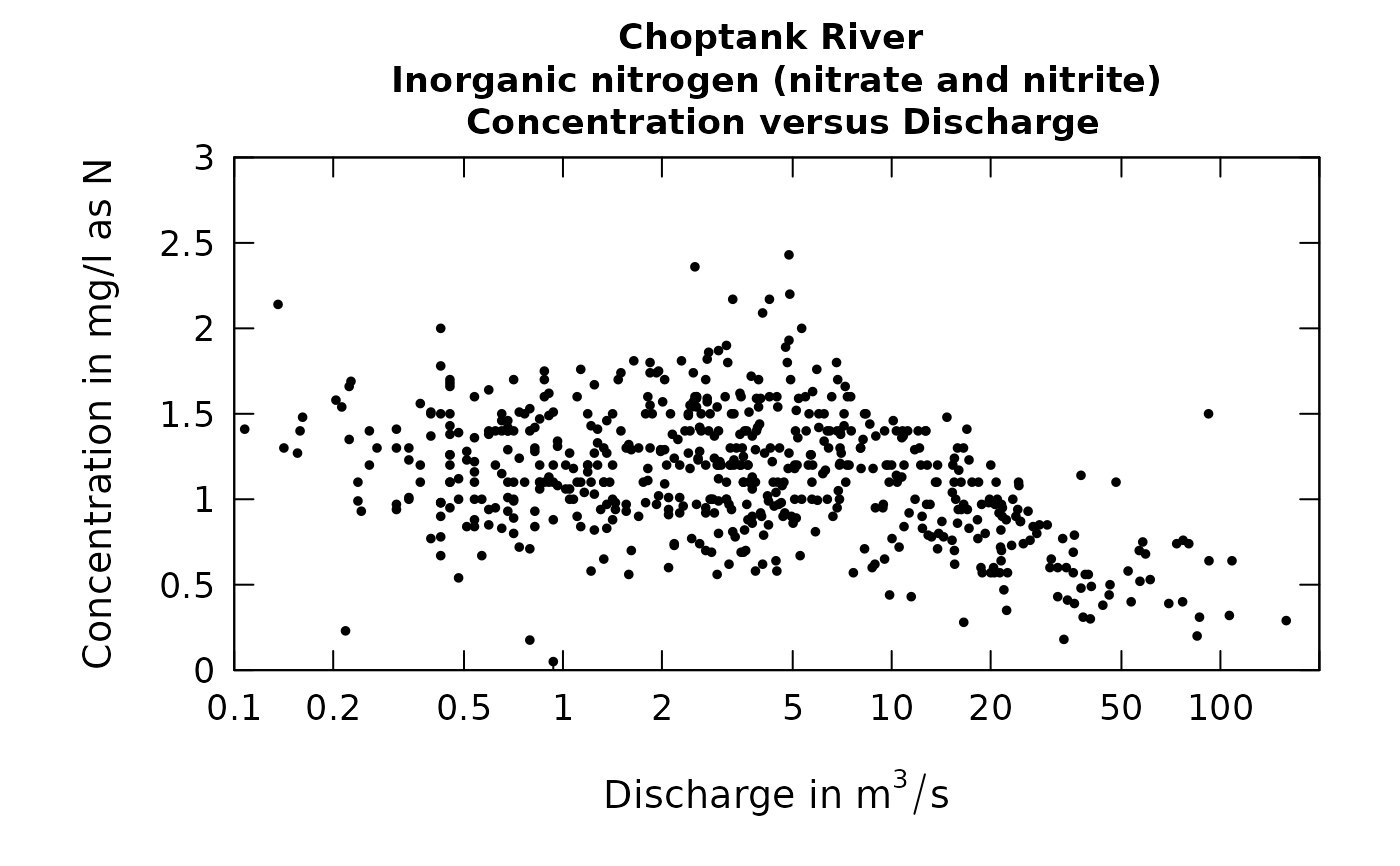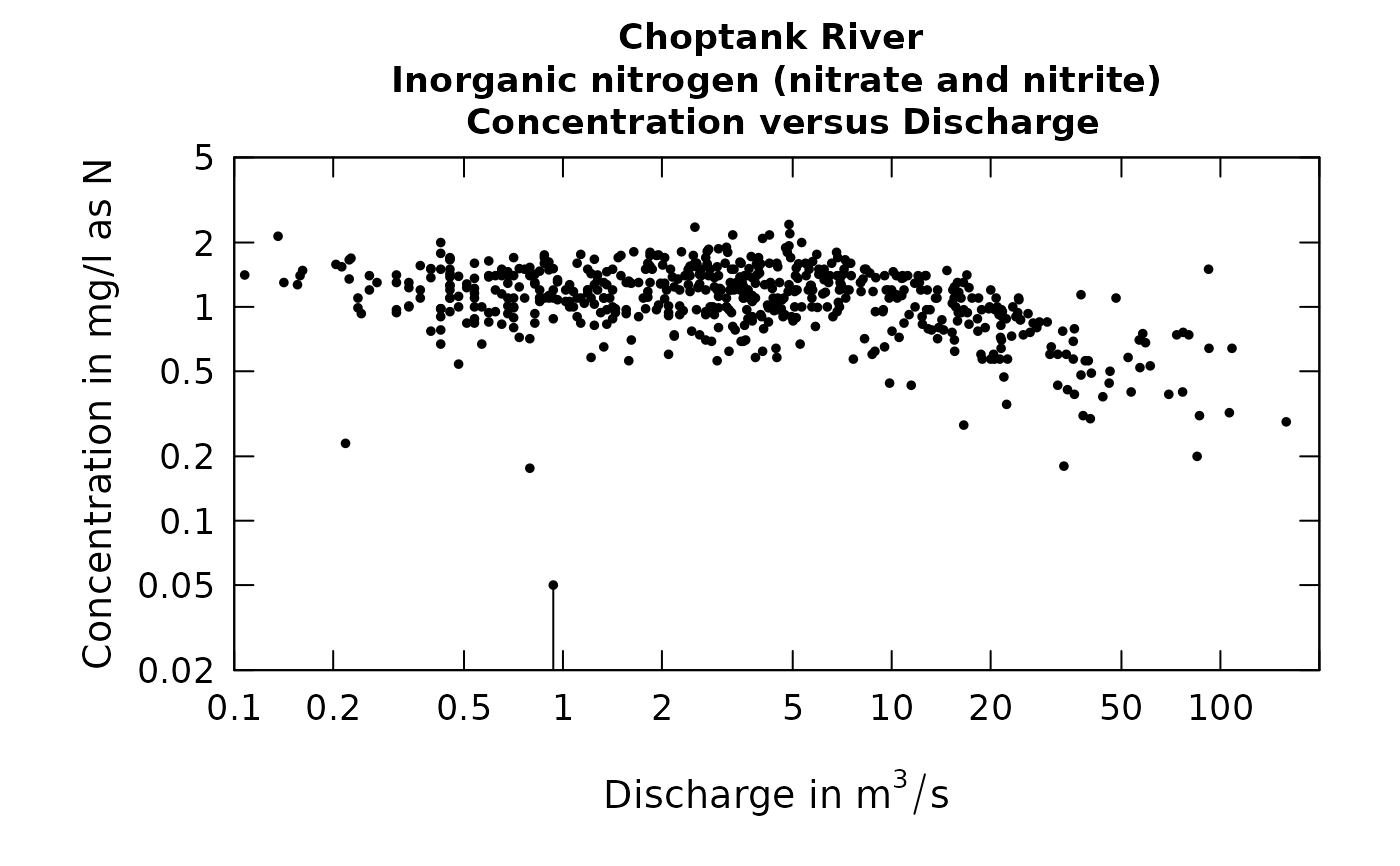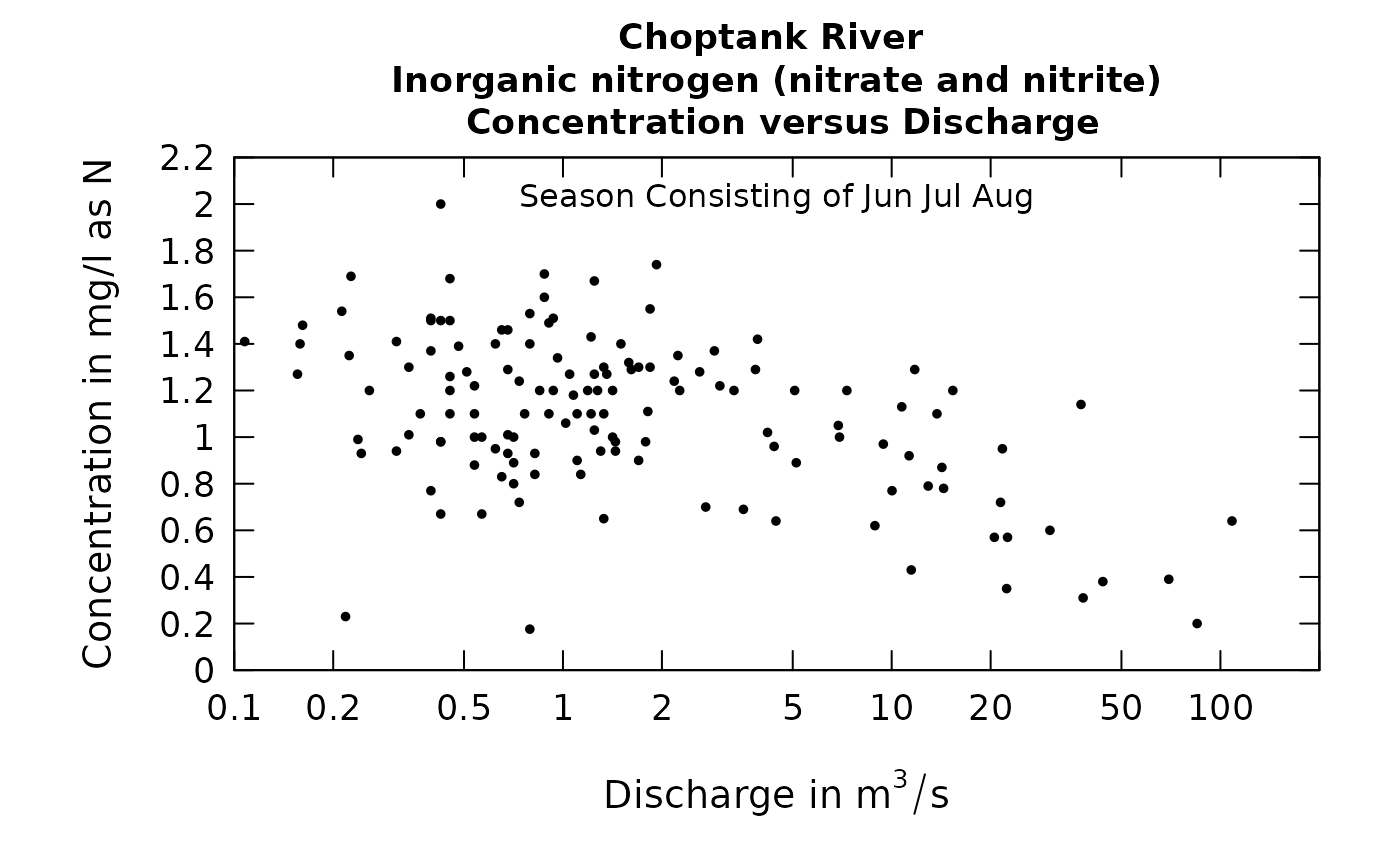Data come from named list, which contains a Sample dataframe with the sample data, and an INFO dataframe with metadata. Discharge is plotted on a log scale.

Although there are a lot of optional arguments to this function, most are set to a logical default.

## Usage

plotConcQ(eList, qUnit = 2, tinyPlot = FALSE, logScale = FALSE,
randomCensored = FALSE, concMax = NA, concMin = NA,
printTitle = TRUE, cex = 0.8, cex.axis = 1.1, cex.main = 1.1,
usgsStyle = FALSE, rmSciX = FALSE, rmSciY = FALSE, customPar = FALSE,
col = "black", lwd = 1, concLab = 1, ...)

## Arguments

eList

named list with at least the Sample and INFO dataframes

qUnit

object of qUnit class printqUnitCheatSheet, or numeric represented the short code, or character representing the descriptive name.

tinyPlot

logical variable, if TRUE plot is designed to be plotted small as part of a multipart figure, default is FALSE.

logScale

logical if TRUE x and y plotted in log axis

randomCensored

logical. Show censored values as randomized.

concMax

number specifying the maximum value to be used on the vertical axis, default is NA (which allows it to be set automatically by the data)

concMin

numeric value for lower limit on concentration shown on the vertical log graph, default is NA (which causes the lower limit to be set automatically, based on the data). This value is ignored for linear scales, using 0 as the minimum value for the concentration axis.

printTitle

logical variable if TRUE title is printed, if FALSE title is not printed (this is best for a multi-plot figure)

cex

numerical value giving the amount by which plotting symbols should be magnified

cex.axis

magnification to be used for axis annotation relative to the current setting of cex

cex.main

magnification to be used for main titles relative to the current setting of cex

usgsStyle

logical option to use USGS style guidelines. Setting this option to TRUE does NOT guarantee USGS compliance. It will only change automatically generated labels.

rmSciX

logical defaults to FALSE, changes x label from scientific to fixed

rmSciY

logical defaults to FALSE, changes y label from scientific to fixed

customPar

logical defaults to FALSE. If TRUE, par() should be set by user before calling this function (for example, adjusting margins with par(mar=c(5,5,5,5))). If customPar FALSE, EGRET chooses the best margins depending on tinyPlot.

col

color of points on plot, see ?par 'Color Specification'

lwd

number line width

concLab

object of concUnit class, or numeric represented the short code, or character representing the descriptive name. By default, this argument sets concentration labels to use either Concentration or Conc (for tiny plots). Units are taken from the eList$INFO$param.units. To use any other words than "Concentration" see vignette(topic = "units", package = "EGRET").

...

arbitrary graphical parameters that will be passed to genericEGRETDotPlot function (see ?par for options)

## Details

The function has two possible ways to plot censored values (e.g. "less-than values").

The default is to plot them as a vertical line that goes from the reporting limit down to the bottom of the graph.

The alternative is to set randomCensored = TRUE. In this case a random value is used for plotting the individual sample value. This random value lies between the reporting limit and zero and it is distributed as a truncated log normal based on the fitted WRTDS model.

The function makeAugmentedSample must be run first if randomCensored = TRUE. Running makeAugmentedSample requires that modelEstimation has already been run.

These random censored values are used to create more readable plots and are not used in any computations about the data set. The random censored values are shown as open circles and the non-censored data are shown as filled dots.

selectDays, genericEGRETDotPlot

## Examples

eList <- Choptank_eList
# Water year:
plotConcQ(eList)plotConcQ(eList, logScale=TRUE)# Graphs consisting of Jun-Aug
eList <- setPA(eList, paStart=6,paLong=3)
plotConcQ(eList)